The GLM Procedure

PROC GLM for Quadratic Least Squares Regression

In polynomial regression, the values of a dependent variable (also called a response variable) are described or predicted in terms of polynomial terms involving one or more independent or explanatory variables. An example of quadratic regression in PROC GLM follows. These data are taken from Draper and Smith (1966, p. 57). Thirteen specimens of 90/10 Cu-Ni alloys are tested in a corrosion-wheel setup in order to examine corrosion. Each specimen has a certain iron content. The wheel is rotated in salt seawater at 30 ft/sec for 60 days. Weight loss is used to quantify the corrosion. The fe variable represents the iron content, and the loss variable denotes the weight loss in milligrams/square decimeter/day in the following DATA step.

title 'Regression in PROC GLM';
data iron;
input fe loss @@;
datalines;
0.01 127.6   0.48 124.0   0.71 110.8   0.95 103.9
1.19 101.5   0.01 130.1   0.48 122.0   1.44  92.3
0.71 113.1   1.96  83.7   0.01 128.0   1.44  91.4
1.96  86.2
;


The SGSCATTER procedure is used in the following statements to request a scatter plot of the response variable versus the independent variable.

proc sgscatter data=iron;
plot loss*fe;
run;
ods graphics off;


The plot in Figure 46.4 displays a strong negative relationship between iron content and corrosion resistance, but it is not clear whether there is curvature in this relationship.

Figure 46.4: Plot of Observed Corrosion Resistance by Iron Content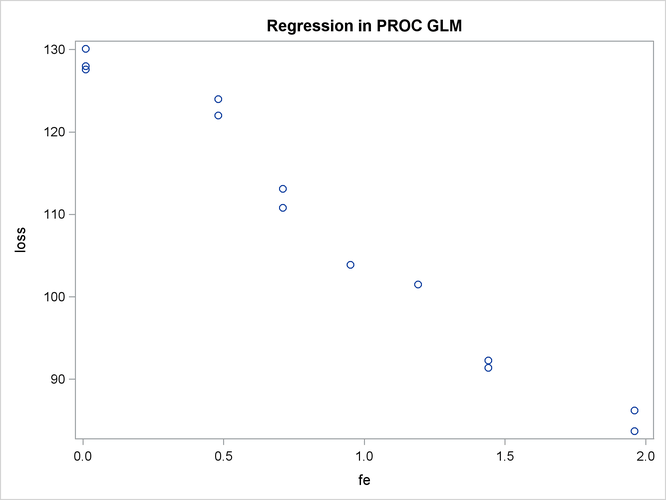The following statements fit a quadratic regression model to the data. This enables you to estimate the linear relationship between iron content and corrosion resistance and to test for the presence of a quadratic component. The intercept is automatically fit unless the NOINT option is specified.

proc glm data=iron;
model loss=fe fe*fe;
run;


The CLASS statement is omitted because a regression line is being fitted. Unlike PROC REG, PROC GLM allows polynomial terms in the MODEL statement.

PROC GLM first displays preliminary information, shown in Figure 46.5, telling you that the GLM procedure has been invoked and stating the number of observations in the data set. If the model involves classification variables, they are also listed here, along with their levels.

Figure 46.5: Data Information

 Regression in PROC GLM

The GLM Procedure

 Number of Observations Read 13 13

Figure 46.6 shows the overall ANOVA table and some simple statistics. The degrees of freedom can be used to check that the model is correct and that the data have been read correctly. The Model degrees of freedom for a regression is the number of parameters in the model minus 1. You are fitting a model with three parameters in this case,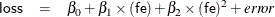so the degrees of freedom are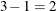. The Corrected Total degrees of freedom are always one less than the number of observations used in the analysis.

Figure 46.6: ANOVA Table

 Regression in PROC GLM

The GLM Procedure

Dependent Variable: loss

Source DF Sum of Squares Mean Square F Value Pr > F
Model 2 3296.530589 1648.265295 164.68 <.0001
Error 10 100.086334 10.008633
Corrected Total 12 3396.616923

R-Square Coeff Var Root MSE loss Mean
0.970534 2.907348 3.163642 108.8154

The R square indicates that the model accounts for 97% of the variation in LOSS. The coefficient of variation (Coeff Var), Root MSE (Mean Square for Error), and mean of the dependent variable are also listed.

The overall F test is significant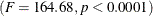, indicating that the model as a whole accounts for a significant amount of the variation in LOSS. Thus, it is appropriate to proceed to testing the effects.

Figure 46.7 contains tests of effects and parameter estimates. The latter are displayed by default when the model contains only continuous variables.

Figure 46.7: Tests of Effects and Parameter Estimates

Source DF Type I SS Mean Square F Value Pr > F
fe 1 3293.766690 3293.766690 329.09 <.0001
fe*fe 1 2.763899 2.763899 0.28 0.6107

Source DF Type III SS Mean Square F Value Pr > F
fe 1 356.7572421 356.7572421 35.64 0.0001
fe*fe 1 2.7638994 2.7638994 0.28 0.6107

Parameter Estimate Standard
Error
t Value Pr > |t|
Intercept 130.3199337 1.77096213 73.59 <.0001
fe -26.2203900 4.39177557 -5.97 0.0001
fe*fe 1.1552018 2.19828568 0.53 0.6107

The t tests provided are equivalent to the Type III F tests. The quadratic term is not significant (p = 0.6107) and thus can be removed from the model; the linear term is significant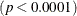. This suggests that there is indeed a straight-line relationship between loss and fe.

Finally, if ODS Graphics is enabled, PROC GLM also displays by default a scatter plot of the original data, as in Figure 46.4, with the quadratic fit overlaid. The following statements, which are the same as the previous analysis but with ODS Graphics enabled, additionally produce Figure 46.8.

ods graphics on;
proc glm data=iron;
model loss=fe fe*fe;
run;
ods graphics off;


Figure 46.8: Plot of Observed and Fit Corrosion Resistance by Iron Content, Quadratic Model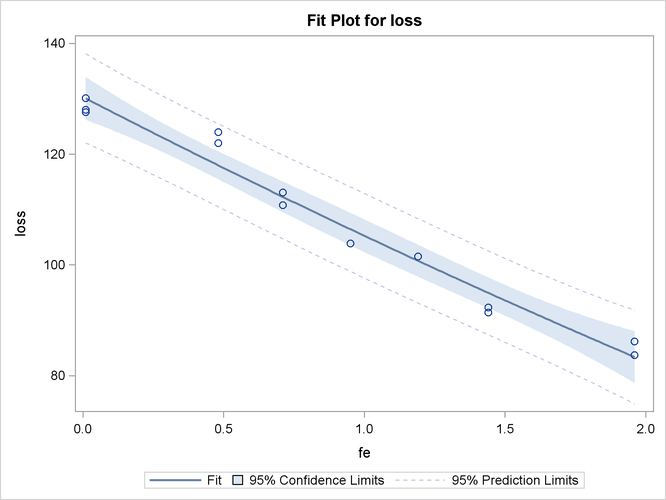The insignificance of the quadratic term in the model is reflected in the fact that the fit is nearly linear.

Fitting the model without the quadratic term provides more accurate estimates for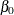and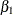. PROC GLM allows only one MODEL statement per invocation of the procedure, so the PROC GLM statement must be issued again. The following statements are used to fit the linear model.

proc glm data=iron;
model loss=fe;
run;


Figure 46.9 displays the output produced by these statements. The linear term is still significant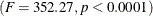. The estimated model is now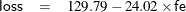Figure 46.9: Linear Model Output

 Regression in PROC GLM

The GLM Procedure

Dependent Variable: loss

Source DF Sum of Squares Mean Square F Value Pr > F
Model 1 3293.766690 3293.766690 352.27 <.0001
Error 11 102.850233 9.350021
Corrected Total 12 3396.616923

R-Square Coeff Var Root MSE loss Mean
0.969720 2.810063 3.057780 108.8154

Source DF Type I SS Mean Square F Value Pr > F
fe 1 3293.766690 3293.766690 352.27 <.0001

Source DF Type III SS Mean Square F Value Pr > F
fe 1 3293.766690 3293.766690 352.27 <.0001

Parameter Estimate Standard
Error
t Value Pr > |t|
Intercept 129.7865993 1.40273671 92.52 <.0001
fe -24.0198934 1.27976715 -18.77 <.0001